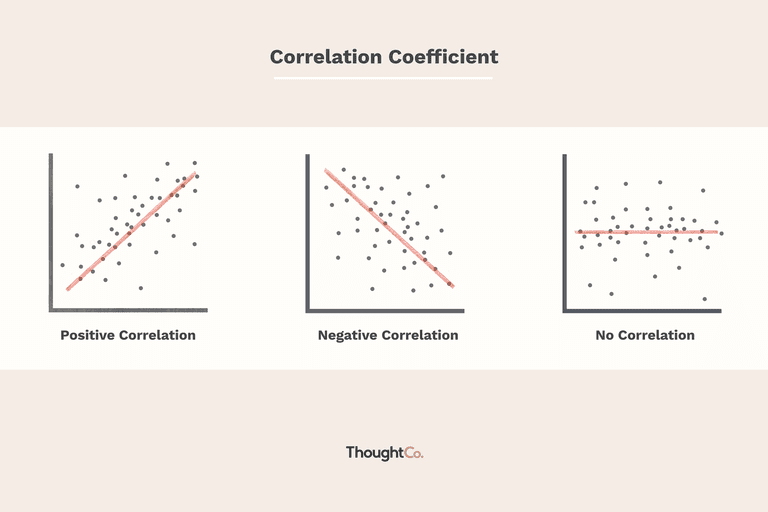/  Top Machine learning interview questions and answers   /  What do you mean by correlation?## What do you mean by correlation?

Correlation means the extent to which the two variables have a linear relationship with each other. It is a statistical technique which can show how strongly variables are related to each other. It is a scaled version of covariance and values ranges from -1 to +1.

It can be calculated as### Ways to detect Correlation between variables:

1. Graphical Method: The pattern of scatter plot indicates the relationship between variables. The relationship can be linear or non-linear.
2. Scatter plot shows the relationship between two variables but does not indicates the strength of relationship amongst them.
3. Non-graphical method: Build the correlation matrix to understand the strength between variables. Correlation varies between -1 and +1.
4. -1: Perfect negative linear correlation
5. +1: Perfect positive linear correlation
6. 0: No correlation